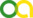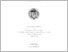Das, Arijit (2018). Design and Analysis of Statistical Learning Algorithms which Control False Discoveries. PhD thesis, Universität zu Köln.Preview
PDF
PhDThesis.pdf - Accepted Version
Bereitstellung unter der CC-Lizenz: Creative Commons Attribution.

Abstract

In this thesis, general theoretical tools are constructed which can be applied to develop ma- chine learning algorithms which are consistent, with fast convergence and which minimize the generalization error by asymptotically controlling the rate of false discoveries (FDR) of features, especially for high dimensional datasets. Even though the main inspiration of this work comes from biological applications, where the data is extremely high dimensional and often hard to obtain, the developed methods are applicable to any general statistical learning problem. In this work, the various machine learning tasks like hypothesis testing, classification, regression, etc are formulated as risk minimization algorithms. This allows such learning tasks to be viewed as optimization problems, which can be solved using first order optimization techniques in case of large data scenarios, while one could use faster converging second order techniques for small to moderately sized data sets. Further, such a formulation allows us to estimate the first order convergence rates of an empirical risk estimator for any arbitrary learning problem, using techniques from large deviation theory. In many scientific applications, robust discovery of factors affecting an outcome or a phe- notype, is more important than the accuracy of predictions. Hence, it is essential to find an appropriate approach to regularize an under-determined estimation problem and thereby control the generalization error. In this work, the use of local probability of false discovery is explored as such a regularization parameter, which forces the optimized solution towards functions with a lower probability to be a false discovery. Again, techniques from large devi- ation theory and the Gibbs principle allow the derivation of an appropriately regularized cost function. These two theoretical results are then used to develop concrete applications. First, the problem of multi-classification is analyzed, which classifies a sample from an arbitrary proba- bility measure into a finite number of categories, based on a given training data set. A general risk functional is derived, which can be used to learn Bayes optimal classifiers controlling the false discovery rate. Secondly, the problem of model selection in the regression context is considered, aiming to select a subset of given regressors which explains most of the observed variation i.e. perform ANOVA. Again, using techniques mentioned above, a risk function is derived which when optimized, controls the rate of false discoveries. This technique is shown to outperform the popular LASSO algorithm, which can be proven to not control the FDR, but only the FWER. Finally, the problem of inferring under-sampled and partially observed non-negative dis- crete random variables is addressed, which has applications to analyzing RNA sequencing data. By assuming infinite divisibility of the underlying random variable, its characterization as being a discrete Compound Poisson Measure (DCP), is derived. This allows construction of a non-parametric Bayesian model of DCPs with a Pitman-Yor Mixture process prior, which is shown to allow for consistent inference under Kullback-Liebler and Renyi divergences even in the under-sampled regime.

Item Type: Thesis (PhD thesis)
Translated title:
TitleLanguage
Design und Analyse statistischer Lernalgorithmen, die False Discoveries kontrollierenGerman
Translated abstract:
AbstractLanguage
In dieser Arbeit werden allgemeine theoretische Methoden entwickelt, die angewendet wer- den können um maschinelle Lernalgorithmen zu generieren die konsistent sind, schnelle Kon- vergenz zeigen und den Generalisierungsfehler minimieren, indem die False Discovery Rate (FDR) insbesondere für hochdimensionale Datensätze gesteuert wird. Obwohl die Hauptin- spiration dieser Arbeit von biologischen Anwendungen herrührt, bei denen die Daten extrem hochdimensional und oft schwer zu erhalten sind, sind die entwickelten Methoden auf alle allgemeinen statistischen Lernprobleme anwendbar. In dieser Arbeit werden die verschiedenen maschinellen Lernaufgaben wie Hypothesen- test, Klassifizierung, Regression usw. als Risikominimierungsalgorithmen formuliert. Auf diese Weise können solche Lernaufgaben als Optimierungsprobleme angesehen werden, die im Fall von großen Datenmengen mit Optimierungstechniken erster Ordnung gelöst werden können, während für kleine bis mittelgroße Datenmengen Techniken zweiter Ordnung mit schnellerer Konvergenz verwendet werden könnten. Darüber hinaus ermöglicht eine solche Formulierung die Schätzung der Konvergenzraten erster Ordnung eines empirischen Risiko- schätzers für jedes beliebige Lernproblem unter Verwendung von Techniken aus der Theorie der großen Abweichungen. In wissenschaftlichen Anwendungen ist eine robuste Detektion von Faktoren, die das Ergebnis beeinflussen, wichtiger als die Genauigkeit von Vorhersagen. Daher ist es wichtig, einen geeigneten Ansatz zu finden, um ein unterbestimmtes Schätzproblem zu regulieren und dadurch den Generalisierungsfehler zu kontrollieren. In dieser Arbeit wird die Verwendung der lokalen Wahrscheinlichkeit einer False Discovery als ein solcher Regularisierungsparam- eter untersucht, der die optimierte Lösung in Richtung von Funktionen mit einer geringeren Wahrscheinlichkeit einer False Discovery zwingt. Auch hier erlauben Techniken der Theorie der großen Abweichungen und des Gibbs-Prinzips die Ableitung einer angemessen regulierten Kostenfunktion. Diese beiden theoretischen Ergebnisse werden anschließend verwendet, um konkrete An- wendungen zu entwickeln. Zunächst wird das Problem der Multi-Klassifikation analysiert, das basierend auf einem gegebenen Trainingsdatensatz eine Stichprobe aus einem beliebi- gen Wahrscheinlichkeitsmaß in eine endliche Anzahl von Kategorien einordnet. Es wird ein allgemeines Risikofunktional abgeleitet, das verwendet werden kann, um optimale Bayes- Klassifikatoren zu lernen, die die False Discovery Rate steuern. Zweitens wird das Problem der Modellauswahl im Regressionskontext betrachtet, das darauf abzielt, eine Untergruppe gegebener Regressoren auszuwählen, die den Großteil der beobachteten Variation erklärt, d. H. ANOVA durchführt. Unter Verwendung der oben er- wähnten Techniken wird wiederum eine Risikofunktion abgeleitet, die, wenn sie optimiert ist, die False Discovery Rate steuert. Diese Methode ermöglicht den Nachweis, dass der häufig verwendete LASSO-Algorithmus nicht FDR sondern nur FWER steuert. Schließlich wird das Problem der Ableitung von unterabgetasteten und teilweise beobachteten nicht-negativen diskreten Zufallsvariablen behandelt, die Anwendungen zur Analyse von RNA- Sequenzierungsdaten haben. Durch Annahme einer unendlichen Teilbarkeit der zugrundeliegen- den Zufallsvariablen wird ihre Charakterisierung als diskretes zusammengesetztes Poisson- Maß (DCP) abgeleitet. Dies ermöglicht die Konstruktion eines nicht-parametrischen Bayes- Modells von DCPs mit einem Pitman-Yor-Mixture-Prozess, der gezeigt hat, dass konsistente Inferenz unter Kullback-Leibler- und Renyi-Divergenzen möglich ist, selbst wenn der Träger unterabgetastet ist.German
Creators:
CreatorsEmailORCIDORCID Put Code
Das, Arijitarijit.das@uni-koeln.deUNSPECIFIEDUNSPECIFIED
URN: urn:nbn:de:hbz:38-98092
Date: 28 May 2018
Language: English
Faculty: Faculty of Mathematics and Natural Sciences
Divisions: Außeruniversitäre Forschungseinrichtungen > MPI for Plant Breeding Research
Subjects: Data processing Computer science
General statistics
Natural sciences and mathematics
Mathematics
Uncontrolled Keywords:
KeywordsLanguage
Machine Learning, Statistical Learning Theory, Hypothesis Testing, Classification, Controlling False Discoveries, Model SelectionEnglish
Date of oral exam: 20 July 2018
Referee:
Tresch, AchimProf. Dr.
Beyer, AndreasProf. Dr.
Refereed: Yes
URI: http://kups.ub.uni-koeln.de/id/eprint/9809View Item